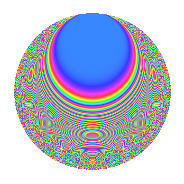# Properties

 Label 201.3.g.bLevel 201 Weight 3 Character orbit 201.g Analytic conductor 5.477 Analytic rank 0 Dimension 84 CM No

# Related objects

## Newspace parameters

 Level: $$N$$ = $$201 = 3 \cdot 67$$ Weight: $$k$$ = $$3$$ Character orbit: $$[\chi]$$ = 201.g (of order $$6$$ and degree $$2$$)

## Newform invariants

 Self dual: No Analytic conductor: $$5.47685331364$$ Analytic rank: $$0$$ Dimension: $$84$$ Relative dimension: $$42$$ over $$\Q(\zeta_{6})$$ Sato-Tate group: $\mathrm{SU}(2)[C_{6}]$

## $q$-expansion

The dimension is sufficiently large that we do not compute an algebraic $$q$$-expansion, but we have computed the trace expansion.

 $$\operatorname{Tr}(f)(q) =$$ $$84q - 6q^{3} + 82q^{4} + 3q^{6} - 10q^{7} + 2q^{9} + O(q^{10})$$ $$\operatorname{Tr}(f)(q) =$$ $$84q - 6q^{3} + 82q^{4} + 3q^{6} - 10q^{7} + 2q^{9} + 6q^{10} - 58q^{12} - 6q^{13} + 78q^{15} - 130q^{16} + 11q^{18} + 72q^{19} - 36q^{21} + 140q^{22} + 72q^{24} - 428q^{25} + 138q^{27} - 8q^{28} + 29q^{30} - 28q^{31} - 30q^{33} - 54q^{34} + 105q^{36} - 24q^{37} - 103q^{39} + 128q^{40} + 252q^{42} + 148q^{43} + 276q^{45} - 146q^{46} + 137q^{48} - 176q^{49} + 15q^{51} - 792q^{52} + 72q^{54} + 28q^{55} - 205q^{57} - 120q^{58} - 64q^{60} + 162q^{61} - 106q^{63} - 604q^{64} - 462q^{66} - 460q^{67} + 101q^{69} + 636q^{70} + 212q^{72} + 238q^{73} + 628q^{75} + 40q^{76} + 96q^{78} + 462q^{79} - 726q^{81} - 344q^{82} + 385q^{84} - 94q^{85} - 91q^{87} + 234q^{88} + 482q^{90} - 536q^{91} + 281q^{93} + 580q^{94} + 40q^{96} + 68q^{97} + 379q^{99} + O(q^{100})$$

## Embeddings

For each embedding $$\iota_m$$ of the coefficient field, the values $$\iota_m(a_n)$$ are shown below.

For more information on an embedded modular form you can click on its label.

Label $$a_{2}$$ $$a_{3}$$ $$a_{4}$$ $$a_{5}$$ $$a_{6}$$ $$a_{7}$$ $$a_{8}$$ $$a_{9}$$ $$a_{10}$$
29.1 −3.31832 1.91583i −2.72368 + 1.25760i 5.34082 + 9.25058i 5.12708i 11.4474 + 1.04501i 5.45809 + 9.45369i 25.6018i 5.83690 6.85059i 9.82263 17.0133i
29.2 −3.24022 1.87074i −1.87878 2.33884i 4.99934 + 8.65911i 4.08787i 1.71228 + 11.0931i −3.91757 6.78542i 22.4439i −1.94039 + 8.78834i −7.64734 + 13.2456i
29.3 −3.10861 1.79476i 1.01703 + 2.82235i 4.44231 + 7.69431i 6.24319i 1.90387 10.5989i 0.163929 + 0.283933i 17.5334i −6.93129 + 5.74084i −11.2050 + 19.4077i
29.4 −3.07823 1.77721i 2.92996 + 0.644471i 4.31698 + 7.47723i 4.16735i −7.87371 7.19099i −2.85784 4.94993i 16.4711i 8.16931 + 3.77655i 7.40628 12.8280i
29.5 −2.93008 1.69168i 1.12713 2.78021i 3.72358 + 6.44943i 3.95114i −8.00582 + 6.23950i 1.40220 + 2.42868i 11.6630i −6.45916 6.26732i 6.68407 11.5772i
29.6 −2.55999 1.47801i −2.72630 + 1.25192i 2.36902 + 4.10327i 7.04577i 8.82964 + 0.824596i 0.952080 + 1.64905i 2.18168i 5.86540 6.82621i −10.4137 + 18.0371i
29.7 −2.37828 1.37310i 0.338320 + 2.98086i 1.77082 + 3.06716i 5.91841i 3.28841 7.55389i 1.61899 + 2.80418i 1.25873i −8.77108 + 2.01697i 8.12658 14.0757i
29.8 −2.32976 1.34509i 2.41314 1.78235i 1.61852 + 2.80337i 6.52133i −8.01945 + 0.906562i −2.84365 4.92535i 2.05247i 2.64647 8.60210i −8.77176 + 15.1931i
29.9 −2.30980 1.33356i −2.73908 1.22370i 1.55678 + 2.69642i 8.02052i 4.69483 + 6.47924i −4.24579 7.35393i 2.36426i 6.00510 + 6.70364i 10.6959 18.5258i
29.10 −2.22999 1.28749i −2.28852 + 1.93976i 1.31525 + 2.27807i 0.0457250i 7.60080 1.37922i −4.62513 8.01096i 3.52645i 1.47465 8.87837i −0.0588703 + 0.101966i
29.11 −2.19394 1.26667i −1.19967 2.74969i 1.20891 + 2.09389i 2.77685i −0.850943 + 7.55223i 5.01703 + 8.68975i 4.00822i −6.12157 + 6.59745i −3.51736 + 6.09224i
29.12 −1.76138 1.01693i 2.83847 + 0.971138i 0.0683121 + 0.118320i 2.80344i −4.01204 4.59708i 5.21582 + 9.03406i 7.85760i 7.11378 + 5.51309i −2.85091 + 4.93792i
29.13 −1.42247 0.821262i 2.43078 1.75821i −0.651057 1.12766i 8.34621i −4.90166 + 0.504680i 1.86864 + 3.23657i 8.70885i 2.81742 8.54764i 6.85443 11.8722i
29.14 −1.34106 0.774260i −2.89057 0.802859i −0.801042 1.38745i 1.39955i 3.25481 + 3.31474i 3.37843 + 5.85161i 8.67494i 7.71083 + 4.64145i 1.08362 1.87688i
29.15 −1.29938 0.750199i 2.00560 + 2.23105i −0.874403 1.51451i 2.91605i −0.932314 4.40358i −6.24309 10.8134i 8.62550i −0.955134 + 8.94917i −2.18761 + 3.78906i
29.16 −1.03426 0.597131i −1.13621 + 2.77651i −1.28687 2.22892i 0.439293i 2.83308 2.19317i −0.664310 1.15062i 7.85076i −6.41804 6.30942i 0.262316 0.454344i
29.17 −0.922015 0.532326i −0.226863 2.99141i −1.43326 2.48248i 3.49902i −1.38323 + 2.87889i −3.66463 6.34732i 7.31045i −8.89707 + 1.35728i 1.86262 3.22615i
29.18 −0.718547 0.414853i 2.98530 0.296617i −1.65579 2.86792i 4.81931i −2.26813 1.02533i −2.30167 3.98661i 6.06647i 8.82404 1.77098i 1.99931 3.46290i
29.19 −0.671554 0.387722i −2.19554 2.04441i −1.69934 2.94335i 9.75594i 0.681761 + 2.22419i −3.81946 6.61550i 5.73727i 0.640779 + 8.97716i −3.78259 + 6.55164i
29.20 −0.481246 0.277848i −1.17888 + 2.75867i −1.84560 3.19668i 8.73533i 1.33382 1.00005i 5.21194 + 9.02735i 4.27397i −6.22049 6.50427i −2.42709 + 4.20384i
See all 84 embeddings
 $$n$$: e.g. 2-40 or 990-1000 Embeddings: e.g. 1-3 or 104.42 Significant digits: Format: Complex embeddings Normalized embeddings Satake parameters Satake angles

## Inner twists

This newform does not have CM; other inner twists have not been computed.

## Hecke kernels

This newform can be constructed as the kernel of the linear operator $$T_{2}^{84} - \cdots$$ acting on $$S_{3}^{\mathrm{new}}(201, [\chi])$$.